Question
A 1200 kg frictionless roller coaster starts from rest at a height of 20 m. What is its kinetic energy when it goes over a hill that is 13 m high?

in kJ

We need at least 10 more requests to produce the answer.

0 / 10 have requested this problem solution

The more requests, the faster the answer.

All students who have requested the answer will be notified once they are available.

#### Earn Coins

Coins can be redeemed for fabulous gifts.

Similar Homework Help Questions
• ### A 1200 kg frictionless roller coaster starts from rest at a height of 17 m. What...

A 1200 kg frictionless roller coaster starts from rest at a height of 17 m. What is its kinetic energy when it goes over a hill that is 10 m high?

• ### a 1200 kg Frictionless roller coaster starts from rest at a height 24 m. what is...

a 1200 kg Frictionless roller coaster starts from rest at a height 24 m. what is the kinetic energy when it goes over a hill that is 12 m high

• ### A 1200-kg frictionless roller coaster starts from rest at a height of 24 m

A 1200-kg frictionless roller coaster starts from rest at a height of 24 m. What is its kinetic energy when it goes over a spot that is 12 m high?

• ### Consider the roller coaster pictured. The coaster car (m= 1000 kg) starts from rest at (1)...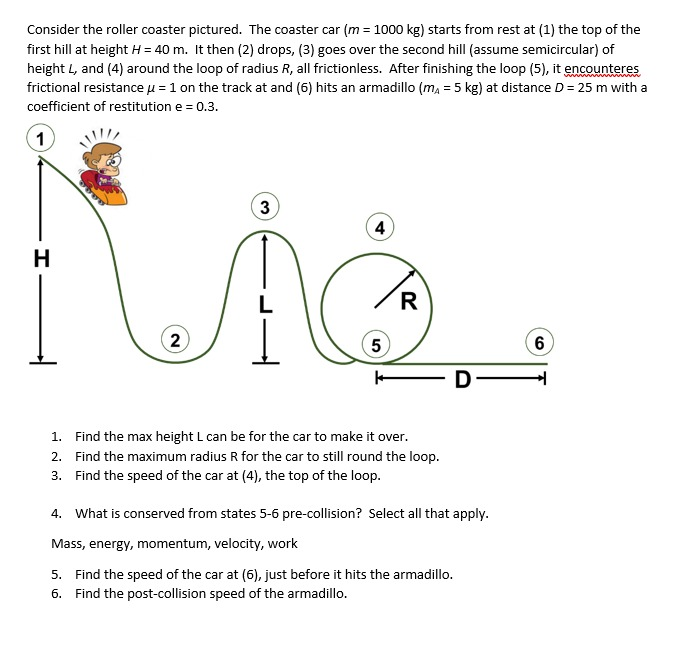Consider the roller coaster pictured. The coaster car (m= 1000 kg) starts from rest at (1) the top of the first hill at height H = 40 m. It then (2) drops, (3) goes over the second hill assume semicircular) of height L, and (4) around the loop of radius R, all frictionless. After finishing the loop (5), it encounteres frictional resistance u = 1 on the track at and (6) hits an armadillo (mA = 5 kg) at distance...

• ### Consider the roller coaster pictured. The coaster car (m= 1000 kg) starts from rest at (1)...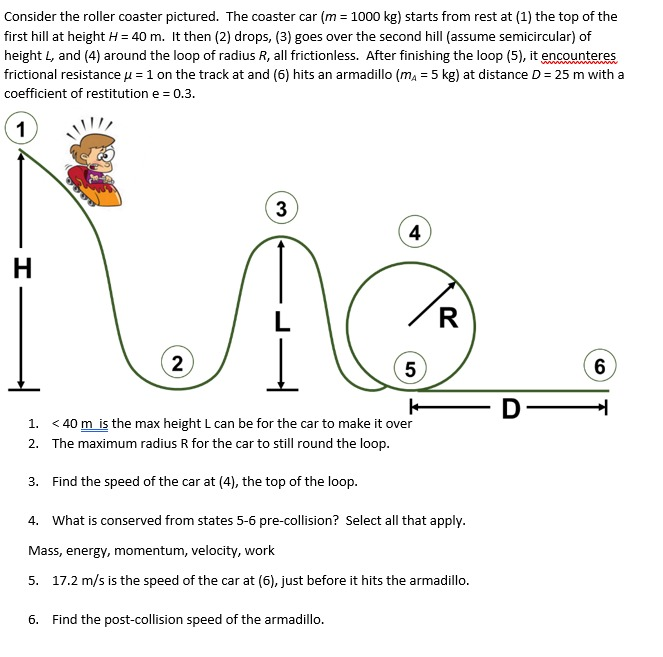Consider the roller coaster pictured. The coaster car (m= 1000 kg) starts from rest at (1) the top of the first hill at height H = 40 m. It then (2) drops, (3) goes over the second hill assume semicircular) of height L, and (4) around the loop of radius R, all frictionless. After finishing the loop (5), it encounteres frictional resistance u = 1 on the track at and (6) hits an armadillo (mA = 5 kg) at distance...

• ### In the figure, a frictionless roller coaster car of mass m = 637 kg tops the...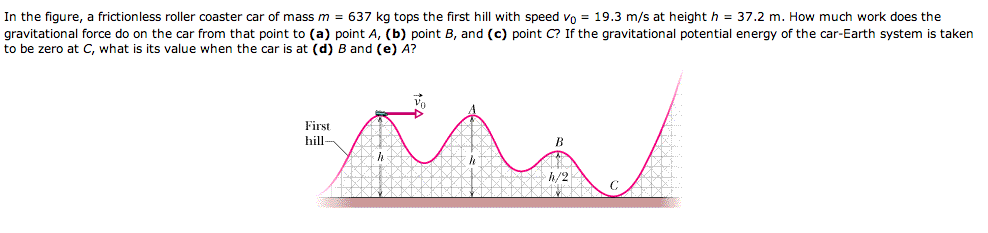In the figure, a frictionless roller coaster car of mass m = 637 kg tops the first hill with speed v0 = 19.3 m/s at height h = 37.2 m. How much work does the gravitational force do on the car from that point to (a) point A, (b) point B, and (c) point C? If the gravitational potential energy of the car-Earth system is taken to be zero at C, what is its value when the car is at...

• ### roller coaster car on the frictionless track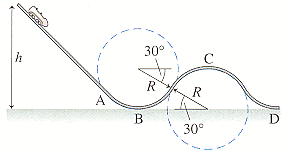A roller coaster car on the frictionless track shown in FigureP10.53 starts from rest at height h. The track is straightuntil point A. Between points A and D,the track consists ofcircle-shaped segments of radius R.(a) What is the maximum heighthmax from which the car can start so as not tofly off the track when going over the hill at point C? Giveyouranswer in terms of the radius R. (Hint: This is a two-part problem.First find vmax at C. Use R...

• ### A roller coaster starts at some height that you do not know. It goes down this...

A roller coaster starts at some height that you do not know. It goes down this hill and then goes up a second hill that is 29.7 m above the first drop. At the top of this hill, it has a speed of 28.5 m/s. So how high was the initial hill?

• ### 4. A roller-coaster car with a mass of 250 kg carries a 75 kg passenger and...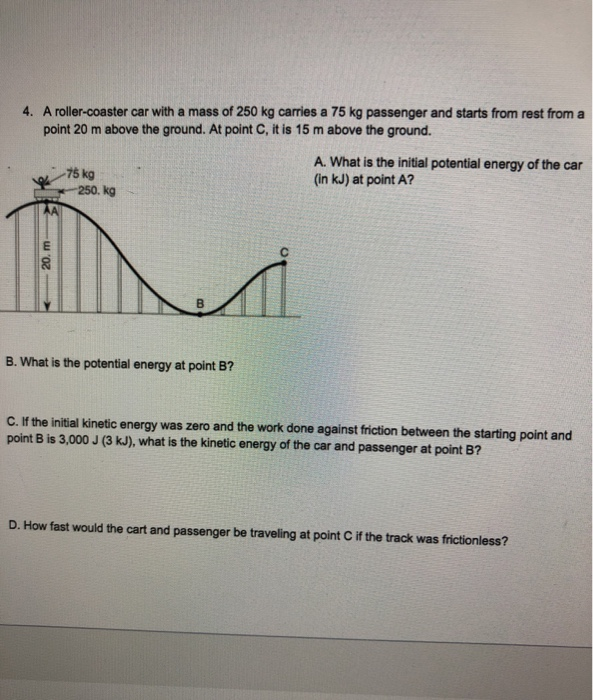4. A roller-coaster car with a mass of 250 kg carries a 75 kg passenger and starts from rest from a point 20 m above the ground. At point C, it is 15 m above the ground. A. What is the initial potential energy of the car (in kJ) at point A? - 75 kg 250. kg B. What is the potential energy at point B? C. If the initial kinetic energy was zero and the work done against friction...

• ### a mass of 200 kg travels down a frictionless roller coaster from a height of 50...

a mass of 200 kg travels down a frictionless roller coaster from a height of 50 m. When it reaches the level ground (height 0 m) it encounters an unstretched horizontal spring with spring constant 10,000 N/m. As the mass comes to a rest how far does it compress the spring?